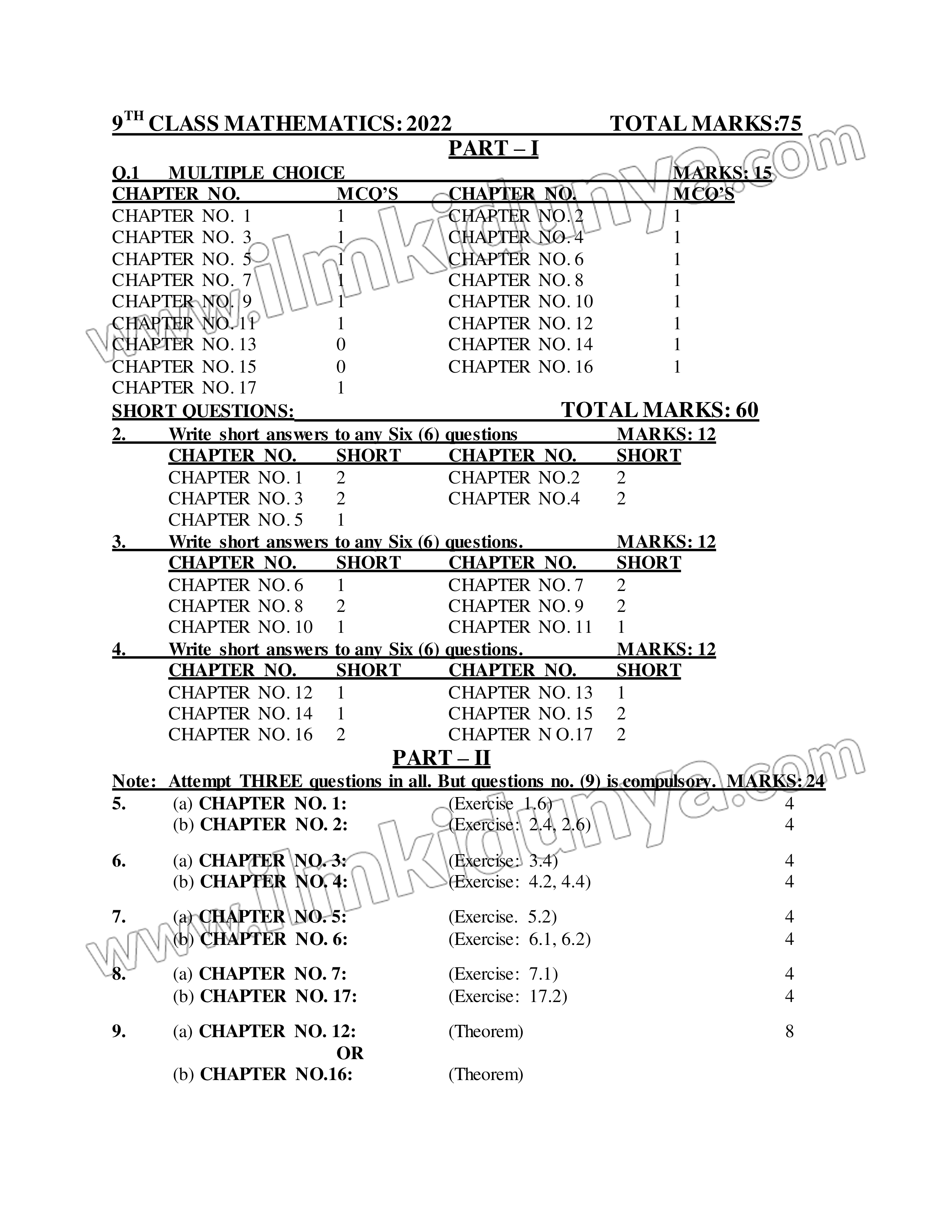×

The candidates that are looking for the 9th class math paper scheme 2023 of the science group are notified that they are at the right portal. The students can check the pairing scheme provided by the board authorities by going through this platform. For the convenience of the students, it is stated that they can access it easily before preparing for the annual exams 2023 of the 9th class.

## Class 9 Mathematics Paper Scheme (Download App For 9th Class Maths Pairing Scheme)

There are a number of the topics included in the syllabus such as Matrices and Determinants, Real and Complex Numbers, Logarithms, Algebraic Expressions, and Algebraic Formulas, Factorization, Algebraic Manipulation, Linear Equations, and Inequalities, Linear Graph  Their Application, Introduction to Coordinate Geometry Descriptive Geometry, Congruent Triangles, Parallelograms and Triangles, Line Bisectors and Angle Bisectors, Sides and Angles of a Triangle, Ratio and Proportion, Pythagoras Theorem, Theorem Related with Area and Practical Geometry-Triangles in 9th class mathematics pairing scheme 2023.The same 9th math pairing scheme is approved for the Punjab Boards such as BISE Lahore, BISE Gujranwala, BISE Sahiwal, BISE Sargodha, BISE Rawalpindi, BISE Faisalabad, BISE DG Khan, BISE Multan, and BISE Bahawalpur. It is advised to the candidates to focus on the main concepts and practice math questions before the exams in order to secure maximum grades. It is furthermore informed to the students that the division of questions for each topic and chapter is also provided that is a part of the 9th syllabus.

9TH CLASS MATHEMATICS: 2023                          TOTAL MARKS:75

PART – I

Q.1      MULTIPLE CHOICE                                                                     MARKS: 15

CHAPTER NO.                    MCQ’S           CHAPTER NO.                    MCQ’S

CHAPTER NO.  1                  1                      CHAPTER NO. 2                   1

CHAPTER NO.  3                  1                      CHAPTER NO. 4                   1

CHAPTER NO.  5                  1                      CHAPTER NO. 6                   1

CHAPTER NO.  7                  1                      CHAPTER NO. 8                   1

CHAPTER NO.  9                  1                      CHAPTER NO. 10                 1

CHAPTER NO. 11                 1                      CHAPTER NO. 12                 1

CHAPTER NO. 13                 0                      CHAPTER NO. 14                 1

CHAPTER NO. 15                 0                      CHAPTER NO. 16                 1

CHAPTER NO. 17                 1

SHORT QUESTIONS:                                             TOTAL MARKS: 60

2.         Write short answers to any Six (6) questions                      MARKS: 12

CHAPTER NO.        SHORT          CHAPTER NO.        SHORT

CHAPTER NO. 1       2                      CHAPTER NO.2        2

CHAPTER NO. 3       2                      CHAPTER NO.4        2

CHAPTER NO. 5       1

3.         Write short answers to any Six (6) questions.                     MARKS: 12

CHAPTER NO.        SHORT          CHAPTER NO.        SHORT

CHAPTER NO. 6       1                      CHAPTER NO. 7       2

CHAPTER NO. 8       2                      CHAPTER NO. 9       2

CHAPTER NO. 10     1                      CHAPTER NO. 11     1

4.         Write short answers to any Six (6) questions.                     MARKS: 12

CHAPTER NO.        SHORT          CHAPTER NO.        SHORT

CHAPTER NO. 12     1                      CHAPTER NO. 13     1

CHAPTER NO. 14     1                      CHAPTER NO. 15     2

CHAPTER NO. 16     2                      CHAPTER N O.17     2

PART – II

Note:   Attempt THREE questions in all. But questions no. (9) is compulsory. MARKS: 24

5.         (a) CHAPTER NO. 1:                      (Exercise 1.6)                                                  4

(b) CHAPTER NO. 2:                     (Exercise: 2.4, 2.6)                                          4

6.         (a) CHAPTER NO. 3:                      (Exercise: 3.4)                                                 4

(b) CHAPTER NO. 4:                     (Exercise: 4.2, 4.4)                                           4

7.         (a) CHAPTER NO. 5:                      (Exercise. 5.2)                                                 4

(b) CHAPTER NO. 6:                     (Exercise: 6.1, 6.2)                                           4

8.         (a) CHAPTER NO. 7:                      (Exercise: 7.1)                                                 4

(b) CHAPTER NO. 17:                   (Exercise: 17.2)                                                4

9.         (a) CHAPTER NO. 12:                    (Theorem)                                                        8

OR

(b) CHAPTER NO.16:                    (Theorem)

## Mathematics Paper Pattern of 9th Class 2023

The paper scheme makes it easy for the students to prepare for the exams by highlighting the important topics. You can find here the paper pattern of mathematics 9th class according to Punjab Board examination 2023 as follows:

Objective Type

Subjective Type

Total Marks

15

Total Marks

60

Time Allowed

20  minutes

Time Allowed

2:10 hrs

Number of MCQs

15

Number of Questions

6

Distribution of Marks

Each MCQ of 1 mark

Distribution of Marks

Attempt 6 short questions out of 9 having 12 marks

Total

15 MCQs

Attempt 6 short questions out of 9 having 12 marks

Attempt 6 short questions out of 9 having 12 marks

Attempt 3 long questions out of 5 having 24 marks. Last question is compulsory. Each question has 8 marks with 2 parts each.

Total

60 marks

Sort By:
•#### Ali Raza

29 Dec 2022

jimma@gmail.com sir

Like
•24 Nov 2022

Like (1)
X

to continue to ilmkidunya.com

X

to continue to ilmkidunya.com

X

to continue to ilmkidunya.com

X